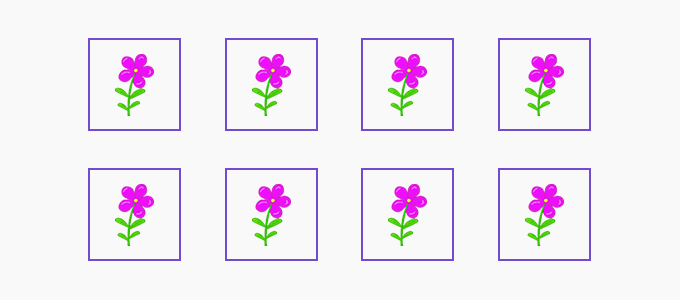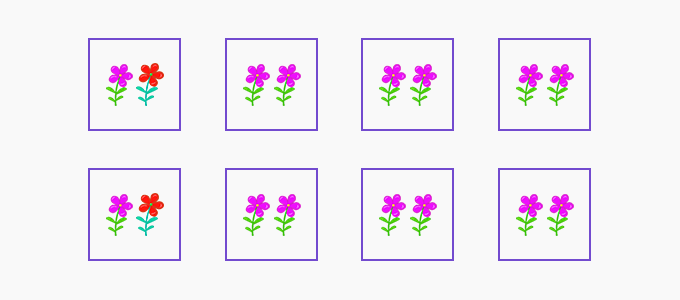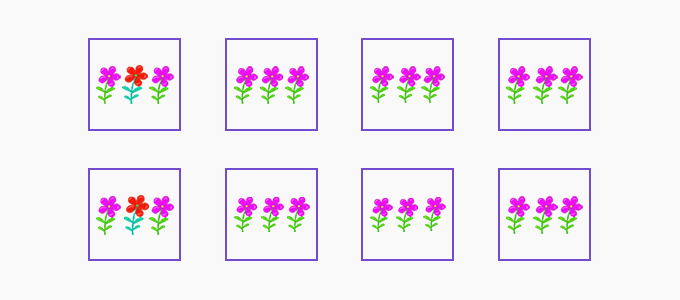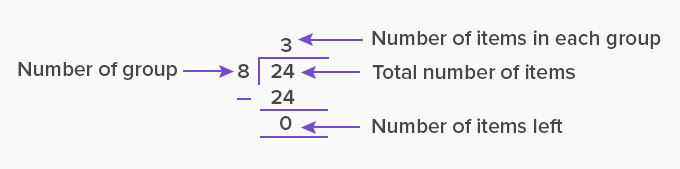# Partitive Division - Definition with Examples

The Complete K-5 Math Learning Program Built for Your Child

• 40 Million Kids

Loved by kids and parent worldwide

• 50,000 Schools

Trusted by teachers across schools

• Comprehensive Curriculum

Aligned to Common Core

What is Partitive Division?

A partitive division problem is one where you know the total number of groups, and are trying to find the number of items in each group.

For example,

There are 24 flowers equally distributed in 8 vases. How many flowers are there in each vase?

Let us see how we can find the answer to this question.

Here, we have eight groups and we have 24 flowers.Let us start by placing 1 flower in each group.On placing 1 flower in each group, we placed 8 flowers in 8 groups.

So, we are left with 24 – 8 = 16 flowers

Again, place 1 flower in each group.Now we have placed another 8 flowers in the 8 groups.

We are left with 16 – 8 = 8 flowers

Again, place 1 flower in each group.After placing the last 8 flowers in the 8 groups, we are left 8 – 8 = 0 flowers.

We see that there are 3 flowers in each group.

We can find the number of items in each group when we know the total number of items and the number of groups by either of the two ways:

We start by subtracting the number of groups from the total number of items. We then subtract the number of groups from the difference obtained. We keep subtracting the number of groups until the remainder is zero or a number smaller than the number of groups.

For example,

24 – 8 = 16

16 – 8 = 8

8 – 8 = 0

The number of times we subtract the number of groups gives the number of items in each group. Here, we subtracted 8 three times. So, the number of items in each group is 3.

2. Division:

To find the number of items in each group, we can directly divide the total number of items (dividend), by the total number of groups (divisor). The quotient obtained is the number of items in each group.Fun Facts The word “partitive” comes from “partition” where the number of items (dividend) is equally partitioned (shared or distributed) into the given number of groups (divisor). Then, we can find the number of items in each group.

Won Numerous Awards & Honors